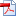## ARTICLES RECENTS / RECENT PAPERS

• Mass-conserving self-similar solutions to coagulation-fragmentation equationspdf

Existence of mass-conserving self-similar solutions with a sufficiently small total mass is proved for a specific class of homogeneous coagulation and fragmentation coefficients. The proof combines a dynamical approach to construct such solutions for a regularised coagulation-fragmentation equation in scaling variables and a compactness method.

• Mass-conserving solutions to coagulation-fragmentation equations with balanced growthpdf

A specific class of coagulation and fragmentation coefficients is considered for which the strength of the coagulation is balanced by that of the multiple fragmentation. Existence and uniqueness of mass-conserving solutions are proved when the initial total mass is sufficiently small.

Colloq. Math., to appear

• Shape derivative of the Dirichlet energy for a transmission problempdf

with Christoph Walker (Hannover)

For a transmission problem in a truncated two-dimensional cylinder located beneath the graph of a function u, the shape derivative of the Dirichlet energy (with respect to u) is shown to be well-defined and is computed. The main difficulties in this context arise from the weak regularity of the domain and the possible non-empty intersection of the graph of u and the transmission interface. The result is applied to establish the existence of a solution to a free boundary transmission problem for an electrostatic actuator.

• Global bounded and unbounded solutions to a chemotaxis system with indirect signal productionpdf

The well-posedness of a chemotaxis system with indirect signal production in a two-dimensional domain is shown, all solutions being global unlike the classical Keller-Segel chemotaxis system. Nevertheless, there is a threshold value Mc of the mass of the first component which separates two different behaviours: solutions are bounded when the mass is below Mc while there are unbounded solutions starting from initial conditions having a mass exceeding Mc. This result extends to arbitrary two-dimensional domains a previous result of Tao & Winkler (2017) obtained for radially symmetric solutions to a simplified version of the model in a ball and relies on a different approach involving a Liapunov functional.

Discrete Contin. Dynam. Systems B, to appear

• Touchdown is the only finite time singularity in a three-dimensional MEMS modelpdf

with Christoph Walker (Hannover)

Touchdown is shown to be the only possible finite time singularity that may take place in a free boundary problem modeling a three-dimensional microelectromechanical system. The proof relies on the energy structure of the problem and uses smoothing effects of the semigroup generated in L1 by the bi-Laplacian with clamped boundary conditions.

• Mass-conserving solutions to the Smoluchowski coagulation equation with singular kernelpdf

with Prasanta Kumar Barik (Roorkee) and Ankik Kumar Giri (Roorkee)

Global weak solutions to the continuous Smoluchowski coagulation equation (SCE) are constructed for coagulation kernels featuring an algebraic singularity for small volumes and growing linearly for large volumes, thereby extending previous results obtained in Norris (1999) and Cueto Camejo & Warnecke (2015). In particular, linear growth at infinity of the coagulation kernel is included and the initial condition may have an infinite second moment. Furthermore, all weak solutions (in a suitable sense) including the ones constructed herein are shown to be mass-conserving, a property which was proved in Norris (1999) under stronger assumptions. The existence proof relies on a weak compactness method in L1 and a by-product of the analysis is that both conservative and non-conservative approximations to the SCE lead to weak solutions which are then mass-conserving.

Proc. Roy. Soc. Edinburgh Sect. A, to appear

• Large time behavior of a two phase extension of the porous medium equationpdf

with Ahmed Ait Hammou Oulhaj (Lille), Clément Cancès (Lille) and Claire Chainais-Hillairet (Lille)

We study the large time behavior of the solutions to a two phase extension of the porous medium equation, which models the so-called seawater intrusion problem. The goal is to identify the self-similar solutions that correspond to steady states of a rescaled version of the problem. We fully characterize the unique steady states that are identified as minimizers of a convex energy and shown to be radially symmetric. Moreover, we prove the convergence of the solution to the time-dependent model towards the unique stationary state as time goes to infinity. We finally provide numerical illustrations of the stationary states and we exhibit numerical convergence rates.